## Producer Field Guide

HGD_Product
Producer Field Guide
HGD_Portfolio_Suite
Producer

The nature of the surface phenomena involved in radar imaging is inherently different from that of visible to infrared (VIS/IR) images. When VIS/IR radiation strikes a surface it is either absorbed, reflected, or transmitted. The absorption is based on the molecular bonds in the (surface) material. Thus, this imagery provides information on the chemical composition of the target.

When radar microwaves strike a surface, they are reflected according to the physical and electrical properties of the surface, rather than the chemical composition. The strength of radar return is affected by slope, roughness, and vegetation cover. The conductivity of a target area is related to the porosity of the soil and its water content. Consequently, radar and VIS/IR data are complementary; they provide different information about the target area. An image in which these two data types are intelligently combined can present much more information than either image by itself.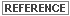See Raster Data and Raster and Vector Data Sources for more information on radar data.

This section describes enhancement techniques that are particularly useful for radar imagery. While these techniques can be applied to other types of image data, this discussion focuses on the special requirements of radar imagery enhancement.For information on Radar Image Enhancement , see Radiometric Enhancement.

Speckle Noise

Speckle noise is commonly observed in radar (microwave or millimeter wave) sensing systems, although it may appear in any type of remotely sensed image utilizing coherent radiation. An active radar sensor gives off a burst of coherent radiation that reflects from the target, unlike a passive microwave sensor that simply receives the low-level radiation naturally emitted by targets.

Like the light from a laser, the waves emitted by active sensors travel in phase and interact minimally on their way to the target area. After interaction with the target area, these waves are no longer in phase. This is because of the different distances they travel from targets, or single versus multiple bounce scattering.

Once out of phase, radar waves can interact to produce light and dark pixels known as speckle noise. Speckle noise must be reduced before the data can be effectively utilized. However, the image processing programs used to reduce speckle noise produce changes in the image.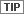Because any image processing done before removal of the speckle results in the noise being incorporated into and degrading the image, you should not rectify, correct to ground range, or in any way resample, enhance, or classify the pixel values before removing speckle noise. Functions using Nearest Neighbor are technically permissible, but not advisable.

Since different applications and different sensors necessitate different speckle removal models, ERDAS IMAGINE Radar module includes several speckle reduction algorithms:

• Mean filter
• Median filter
• Lee-Sigma filter
• Local Region filter
• Lee filter
• Frost filter
• Gamma-MAP filter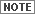Speckle noise in radar images cannot be completely removed. However, it can be reduced significantly.

These filters are described in the following sections:

Mean Filter

Mean filter is a simple calculation. The pixel of interest (center of window) is replaced by the arithmetic average of all values within the moving window. This filter does not remove the aberrant (speckle) value; it averages it into the data.

In theory, a bright and a dark pixel within the same window would cancel each other out. This consideration would argue in favor of a large moving window size (for example, 7 × 7). However, averaging results in a loss of detail, which argues for a small moving window size.

In general, this is the least satisfactory method of speckle reduction. It is useful for applications where loss of resolution is not a problem.

Median Filter

A better way to reduce speckle, but still simplistic, is Median filter. This filter operates by arranging all DN values in sequential order within the window that you define. The pixel of interest is replaced by the value in the center of this distribution. A Median filter is useful for removing pulse or spike noise. Pulse functions of less than one-half of the moving window width are suppressed or eliminated. In addition, step functions or ramp functions are retained.

The effect of Mean and Median filters on various signals is shown (for one dimension) in the following figure.

Effects of Mean and Median Filters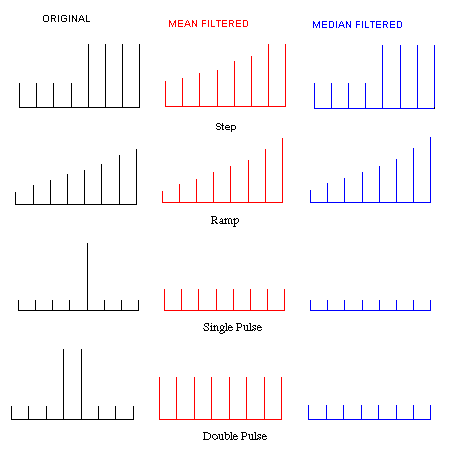Median filter is useful for noise suppression in any image. It does not affect step or ramp functions; it is an edge preserving filter (Pratt, 1991). It is also applicable in removing pulse function noise, which results from the inherent pulsing of microwaves. An example of Median filter is the removal of dead-detector striping, as found in Landsat 4 TM data (Crippen, 1989a).

Local Region Filter

Local Region filter divides the moving window into eight regions based on angular position (North, South, East, West, NW, NE, SW, and SE). The figure below shows a 5 × 5 moving window and the regions of the Local Region filter.

Regions of Local Region Filter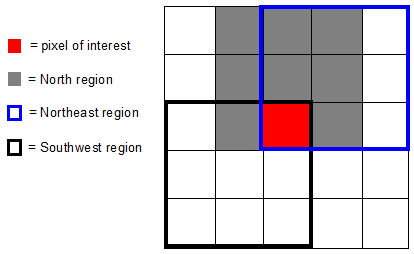For each region, the variance is calculated as follows: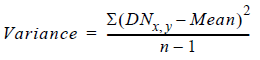Source: Nagao and Matsuyama, 1978

The algorithm compares the variance values of the regions surrounding the pixel of interest. The pixel of interest is replaced by the mean of all DN values within the region with the lowest variance (that is, the most uniform region). A region with low variance is assumed to have pixels minimally affected by wave interference, yet very similar to the pixel of interest. A region of low variance is probably such for several surrounding pixels.

The result is that the output image is composed of numerous uniform areas, the size of which is determined by the moving window size. In practice, this filter can be utilized sequentially 2 or 3 times, increasing the moving window size. The resultant image is an appropriate input to a classification application.

Lee-Sigma and Lee Filters

Sigma and Lee filters use the statistical distribution of DN values within the moving window to estimate what the pixel of interest should be.

Lee filter is based on the assumption that the mean and variance of the pixel of interest is equal to the local mean and variance of all pixels within the user-selected moving window.

A mathematical model in which the noise is multiplicative is also assumed. Implementation of this (and the Lee-Sigma filter) requires you to enter an estimated value for sigma (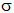), the coefficient of variation within the scene of interest. Calculations based on a Rayleigh distribution model yield aof 0.26 for 4-look radar data. This value is consistent with those derived from actual data (Seasat, SIR-B). A sigma value () for any data set may be derived as: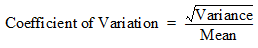Speckle in imaging radar can be mathematically modeled as multiplicative noise with a mean of 1. The standard deviation of the noise can be mathematically defined as: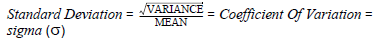Coefficient of variation, as a scene-derived parameter, is used as an input parameter in the Sigma and Lee filters. It is also useful in evaluating and modifying VIS/IR data for input to a 4-band composite image, or in preparing a 3-band ratio color composite (Crippen, 1989a).

Imaging radar data noise is assumed to follow a Gaussian distribution. This yields a theoretical value for Standard Deviation (SD) of 0.52 for 1-look radar data and SD = 0.26 for 4-look radar data.

The table below lists theoretical coefficient of variation values for various look-average radar scenes:

 # of Looks (scenes) Coefficient of Variation Value 1 0.52 2 0.37 3 0.30 4 0.26 6 0.21 8 0.18

Lee filters are based on the assumption that the mean and variance of the pixel of interest are equal to the local mean and variance of all pixels within the moving window you select.

The actual calculation used for the Lee filter is: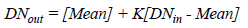Where:

Mean = average of pixels in a moving window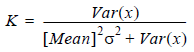The variance of x [Var (x)] is defined as: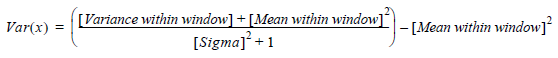Source: Lee, 1981

Lee-Sigma filter is based on the probability of a Gaussian distribution. It is assumed that 95.5% of random samples are within a 2 standard deviation (2 sigma) range. This noise suppression filter replaces the pixel of interest with the average of all DN values within the moving window that fall within the designated range.

As with all the radar speckle filters, you must specify a moving window size. The center pixel of the moving window is the pixel of interest.

As with Statistics filter, a coefficient of variation specific to the data set must be entered. Finally, you must specify how many standard deviations to use (2, 1, or 0.5) to define the accepted range.

The statistical filters (Lee-Sigma and Statistics) are logically applicable to any data set for preprocessing. Any sensor system has various sources of noise, resulting in a few erratic pixels. In VIS/IR imagery, most natural scenes are found to follow a normal distribution of DN values, thus filtering at 2 standard deviations should remove this noise. This is particularly true of experimental sensor systems that frequently have significant noise problems.

These speckle filters can be used iteratively. You must view and evaluate the resultant image after each pass (the data histogram is useful for this), and then decide if another pass is appropriate and what parameters to use on the next pass. For example, three passes of the Lee-Sigma filter with the following parameters are very effective when used with any type of data:

 Pass Sigma Value Sigma Multiplier Window Size 1 0.26 0.5 3 × 3 2 0.26 1 5 × 5 3 0.26 2 7 × 7

Similarly, there is no reason why successive passes must be of the same filter. The following sequence is useful prior to a classification:

 Filter Pass Sigma Value Sigma Multiplier Window Size Lee 1 0.26 NA 3 × 3 Lee 2 0.26 NA 5 × 5 Local Region 3 NA NA 5 × 5 or 7 × 7With all speckle reduction filters there is a playoff between noise reduction and loss of resolution. Each data set and each application have a different acceptable balance between these two factors. The ERDAS IMAGINE filters have been designed to be versatile and gentle in reducing noise (and resolution).

Frost Filter

Frost filter is a minimum mean square error algorithm that adapts to the local statistics of the image. The local statistics serve as weighting parameters for the impulse response of the filter (moving window). This algorithm assumes that noise is multiplicative with stationary statistics.

The formula used is: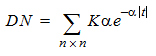Where: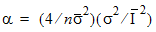and

K = normalization constant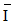=  local mean= local variance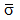= image coefficient of variation value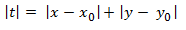n = moving window size

Source: Lopes et al, 1990

Gamma-MAP Filter

Maximum A Posteriori (MAP) filter attempts to estimate the original pixel DN, which is assumed to lie between the local average and the degraded (actual) pixel DN. MAP logic maximizes the a posteriori probability density function with respect to the original image.

Many speckle reduction filters (for example, Lee, Lee-Sigma, Frost) assume a Gaussian distribution for the speckle noise. Recent work has shown this to be an invalid assumption. Natural vegetated areas have been shown to be more properly modeled as having a Gamma distributed cross section. This algorithm incorporates this assumption. The exact formula used is the cubic equation: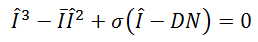Where: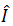= sought value= local mean

DN= input value= original image variance

Source: Frost et al, 1982

Edge Detection

Edge and line detection are important operations in digital image processing. For example, geologists are often interested in mapping lineaments, which may be fault lines or bedding structures. For this purpose, edge and line detection are major enhancement techniques.

In selecting an algorithm, it is first necessary to understand the nature of what is being enhanced. Edge detection could imply amplifying an edge, a line, or a spot, as shown in the figure below.

One-dimensional, Continuous Edge, and Line Models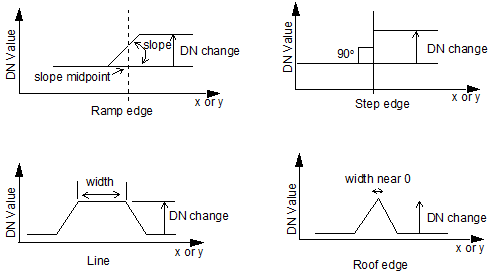• Ramp edge—an edge modeled as a ramp, increasing in DN value from a low to a high level, or vice versa. Distinguished by DN change, slope, and slope midpoint.
• Step edge—a ramp edge with a slope angle of 90 degrees.
• Line—a region bounded on each end by an edge; width must be less than the moving window size.
• Roof edge—a line with a width near zero.

The models in the figure above represent ideal theoretical edges. However, real data values vary to produce a more distorted edge due to sensor noise or vibration, as shown in the figure below. There are no perfect edges in raster data, hence the need for edge detection algorithms.

Noisy Edge Superimposed on an Ideal Edge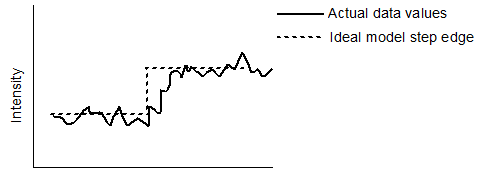Edge detection algorithms can be broken down into 1st-order derivative and 2nd-order derivative operations. The figure below shows ideal one-dimensional edge and line intensity curves with the associated 1st-order and 2nd-order derivatives.

Edge and Line Derivatives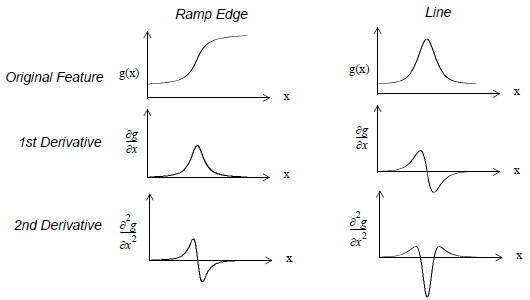The 1st-order derivative kernel or kernels derive from the simple Prewitt kernel: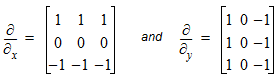The 2nd-order derivative kernel or kernels derive from Laplacian operators: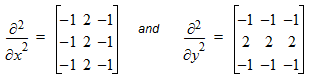1st-Order Derivatives ( Prewitt)

ERDAS IMAGINE Radar module utilizes sets of template matching operators. These operators approximate to the eight possible compass orientations (North, South, East, West, Northeast, Northwest, Southeast, Southwest). The compass names indicate the slope direction creating maximum response. ( Gradient kernels with zero weighting, that is, the sum of the kernel coefficient is zero, have no output in uniform regions.) The detected edge is orthogonal to the gradient direction.

To avoid positional shift, all operating windows are odd number arrays, with the center pixel being the pixel of interest. Extension of the 3 × 3 impulse response arrays to a larger size is not clear cut—different authors suggest different lines of rationale. For example, it may be advantageous to extend the 3-level (Prewitt, 1970) to: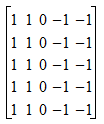or the following might be beneficial: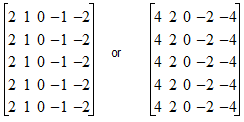Larger template arrays provide a greater noise immunity, but are computationally more demanding.

Zero-Sum Filters

A common type of edge detection kernel is a zero-sum filter. For this type of filter, the coefficients are designed to add up to zero. Following are examples of two zero-sum filters: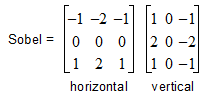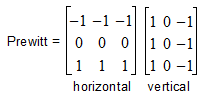Prior to edge enhancement, you should reduce speckle noise by using the ERDAS IMAGINE Radar Speckle Suppression function.

2nd-Order Derivatives (Laplacian Operators)

The second category of edge enhancers is 2nd-order derivative or Laplacian operators. These are best for line (or spot) detection as distinct from ramp edges. ERDAS IMAGINE Radar module offers two such arrays:

Unweighted line: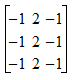Weighted line: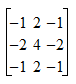Source: Pratt, 1991

Some researchers have found that a combination of 1st- and 2nd-order derivative images produces the best output. See Eberlein and Weszka (Eberlein and Weszka, 1975) for information about subtracting the 2nd-order derivative (Laplacian) image from the 1st-order derivative image (gradient).

Texture

According to Pratt (Pratt, 1991), "Many portions of images of natural scenes are devoid of sharp edges over large areas. In these areas the scene can often be characterized as exhibiting a consistent structure analogous to the texture of cloth. Image texture measurements can be used to segment an image and classify its segments."

As an enhancement, texture is particularly applicable to radar data, although it may be applied to any type of data with varying results. For example, it has been shown (Blom and Daily, 1982) that a three-layer variance image using 15 × 15, 31 × 31, and 61 × 61 windows can be combined into a three-color RGB (red, green, blue) image that is useful for geologic discrimination. The same could apply to a vegetation classification.

You could also prepare a three-color image using three different functions operating through the same (or different) size moving windows. However, each data set and application would need different moving window sizes and texture measures to maximize the discrimination.

While texture analysis has been useful in the enhancement of VIS/IR image data, it is showing even greater applicability to radar imagery. In part, this stems from the nature of the imaging process itself.

The interaction of the radar waves with the surface of interest is dominated by reflection involving the surface roughness at the wavelength scale. In VIS/IR imaging, the phenomena involved is absorption at the molecular level. Also, as we know from array-type antennae, radar is especially sensitive to regularity that is a multiple of its wavelength. This provides for a more precise method for quantifying the character of texture in a radar return.

The ability to use radar data to detect texture and provide topographic information about an image is a major advantage over other types of imagery where texture is not a quantitative characteristic.

The texture transforms can be used in several ways to enhance the use of radar imagery. Adding the radar intensity image as an additional layer in a (vegetation) classification is fairly straightforward and may be useful. However, the proper texture image (function and window size) can greatly increase the discrimination. Using known test sites, one can experiment to discern which texture image best aids the classification. For example, the texture image could then be added as an additional layer to the TM bands.

Texture Analysis Algorithms

While texture has typically been a qualitative measure, it can be enhanced with mathematical algorithms. Many algorithms appear in literature for specific applications (Haralick, 1979; Iron and Petersen, 1981).

The algorithms incorporated into ERDAS IMAGINE are those which are applicable in a wide variety of situations and are not computationally over-demanding. This later point becomes critical as the moving window size increases. Research has shown that very large moving windows are often needed for proper enhancement. For example, Blom (Blom and Daily, 1982) uses up to a 61 × 61 window.

Four algorithms are currently utilized for texture enhancement in ERDAS IMAGINE:

• mean Euclidean distance (1st-order)
• variance (2nd-order)
• skewness (3rd-order)
• kurtosis (4th-order)

Mean Euclidean Distance

These algorithms are shown below (Iron and Petersen, 1981):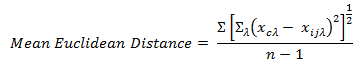Where: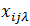=  DN value for spectral band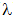and pixel (i,j) of a multispectral image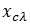=  DN value for spectral bandof a window’s center pixel

n =  number of pixels in a window

Variance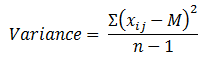Where: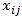=  DN value of pixel (i,j)

n  =  number of pixels in a window

M  =  Mean of the moving window, where: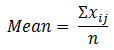Skewness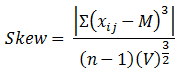Where:= DN value of pixel (i,j)

n = number of pixels in a window

M = Mean of the moving window (see Variance Mean)

V = Variance (see Variance formula)

Kurtosis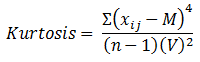Where:= DN value of pixel (i,j)

n = number of pixels in a window

M = Mean of the moving window (see Variance Mean)

V = Variance (see Variance formula)Texture analysis is available in ERDAS IMAGINE Spatial Enhancement and in Radar Utilities.

• imperfections in the transmit and receive pattern of the radar antenna
• errors due to the coherent pulse (that is, speckle)
• inherently stronger signal from a near range (closest to the sensor flight path) than a far range (farthest from the sensor flight path) target

Many imaging radar systems use a single antenna that transmits the coherent radar burst and receives the return echo. However, no antenna is perfect; it may have various lobes, dead spots, and imperfections. This causes the received signal to be slightly distorted radiometrically. In addition, range fall-off causes far range targets to be darker (less return signal).

These two problems can be addressed by adjusting the average brightness of each range line to a constant—usually the average overall scene brightness (Chavez and Berlin, 1986). This requires that each line of constant range be long enough to reasonably approximate the overall scene brightness, as shown in the figure below. This approach is generic; it is not specific to any particular radar sensor.

Adjust Brightness function in ERDAS IMAGINE works by correcting each range line average. For this to be a valid approach, the number of data values must be large enough to provide good average values. Be careful not to use too small an image. This depends upon the character of the scene itself.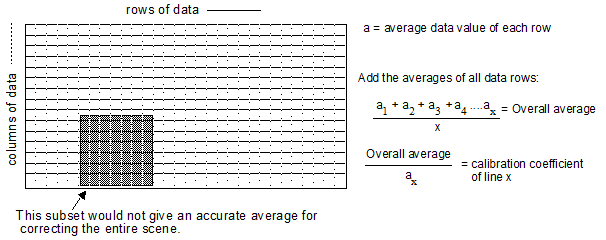Range Lines/Lines of Constant Range

Lines of constant range are not the same thing as range lines:

• Range lines—lines that are perpendicular to the flight of the sensor
• Lines of constant range—lines that are parallel to the flight of the sensor
• Range direction—same as range lines

Because radiometric errors are a function of the imaging geometry, the image must be correctly oriented during the correction process. For the algorithm to correctly address the data set, you must tell ERDAS IMAGINE whether the lines of constant range are in columns or rows in the displayed image.

The figure below shows the lines of constant range in columns, parallel to the sides of the display screen:

Range Lines compared to Lines of Constant Range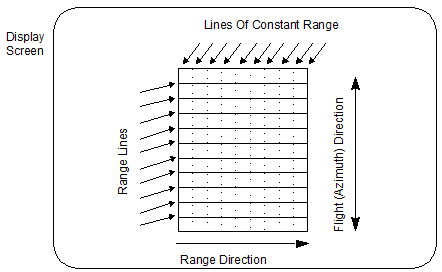As aforementioned, the phenomena involved in radar imaging is quite different from that in VIS/IR imaging. Because these two sensor types give different information about the same target (chemical compared to physical), they are complementary data sets. If the two images are correctly combined, the resultant image conveys both chemical and physical information and could prove more useful than either image alone.

The methods for merging radar and VIS/IR data are still experimental and open for exploration. The following methods are suggested for experimentation:

• Codisplaying in a View
• RGB to IHS transforms
• Principal components transform
• Multiplicative

The ultimate goal of enhancement is not mathematical or logical purity; it is feature extraction. There are currently no rules to suggest which options yield the best results for a particular application; you must experiment. The option that proves to be most useful depends upon the data sets (both radar and VIS/IR), your experience, and your final objective.

Codisplaying

The simplest and most frequently used method of combining radar with VIS/IR imagery is codisplaying on an RGB color monitor. In this technique, the radar image is displayed with one (typically the red) gun, while the green and blue guns display VIS/IR bands or band ratios. This technique follows from no logical model and does not truly merge the two data sets.Use the Viewer for this type of merge. Set the color channels to display the different layers.

RGB to IHS Transforms

Another common technique uses the RGB to IHS transforms. In this technique, an RGB color composite of bands (or band derivatives, such as ratios) is transformed into IHS color space. The intensity component is replaced by the radar image, and the scene is reverse transformed. This technique integrally merges the two data types.For more information, see RGB to IHS.

Principal Components Transform

A similar image merge involves utilizing the PC transformation of the VIS/IR image. With this transform, more than three components can be used. These are converted to a series of principal components. The first PC, PC-1, is generally accepted to correlate with overall scene brightness. This value is replaced by the radar image and the reverse transform is applied.For more information, see Principal Components Analysis.

Multiplicative

A final method to consider is the multiplicative technique. This requires several chromatic components and a multiplicative component, which is assigned to the image intensity. In practice, the chromatic components are usually band ratios or PCs; the radar image is input multiplicatively as intensity (Croft (Holcomb), 1993).

The two sensor merge models using transforms to integrate the two data sets (PC and RGB to IHS) are based on the assumption that the radar intensity correlates with the intensity that the transform derives from the data inputs. However, the logic of mathematically merging radar with VIS/IR data sets is inherently different from the logic of the SPOT/TM merges, as discussed in Resolution Merge. It cannot be assumed that the radar intensity is a surrogate for, or equivalent to, the VIS/IR intensity. The acceptability of this assumption depends on the specific case.

For example, Landsat TM imagery is often used to aid in mineral exploration. A common display for this purpose is RGB = TM5/TM7, TM5/TM4, TM3/TM1; the logic being that if all three ratios are high, the sites suited for mineral exploration are bright overall. If the target area is accompanied by silicification, which results in an area of dense angular rock, this should be the case. However, if the alteration zone is basaltic rock to kaolinite/alunite, then the radar return could be weaker than the surrounding rock. In this case, radar would not correlate with high 5/7, 5/4, 3/1 intensity and the substitution would not produce the desired results (Croft (Holcomb), 1993).